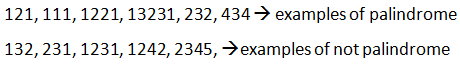# Palindrome string (number)

StringViews 1075

## Check whether the given string is palindrome or not. Given string is form of positive number.

Palindrome: If we reverse the string if it looks the same then it is called as palindrome.

### ExamplesTime complexity : O(n)

## Algorithm

a. Copy the number and store it in temp.

b. Create a new variable reverse, reverse = 0.

c.  reverse = reverse * 10, divide temp with 10 and add remainder to reverse.(reverse = reverse + temp)

d. And update temp = temp/10, do this until temp = 0

e. Finally if input number = reverse, print is palindrome.

f. Else, print not palindrome.

## C++ Program

```#include <bits/stdc++.h>

using namespace std;

int main()
{
//n is input number
int n, reverse = 0, temp;
cout<<"Enter a number to check if it is a palindrome or not: ";
cin>>n;
temp = n;
//reverse is reverse ot it
while (temp != 0)
{
reverse = reverse * 10;
reverse = reverse + temp;
temp    = temp/10;
}

if (n == reverse)
{
cout<<n<<" is a palindrome number";
}
else
{
cout<<n<<" is not a palindrome number";
}
return 0;
}```

Translate »